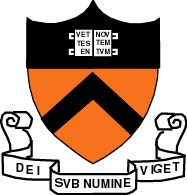Course Summary

Can the mechanism of learning be automated and implemented by a machine?
In this course we formally define and study various models that have been proposed for learning. The course will present and contrast the statistical, computational and game-theoretic models for learning. We will present and rigorously analyze some of the most successful algorithms in machine learning that are extensively used today. Likely topics include: intro to statistical learning theory and bounds on the number of random examples needed to learn; learning in adversarial settings and the on-line learning model; using convex optimization to model and solve learning problems; learning with partial observability; how to boost the accuracy of a weak learning algorithm; universal portfolio selection; learning in games; unsupervised learning; spectral methods in learning.

Lectures: Tue + Thu, 11:00-12:20 in CS building, room 105.

Professor: Elad Hazan, CS building 407, Reception hours: Tue 14-15, Fri 10:30-11:30, or by appointment.

Teaching Assistants:
Brian Bullins, CS building second floor, Reception hours: Wednesdays 14:00-15:00
Jason Altschuler , CS building tea room, Reception hours: Fridays 14:30-16:00

Requirements: This is a graduate-level course that requires significant mathematical background.
Required background: probability, discrete math, calculus, analysis, linear algebra, algorithms and data structures, theory of computation / complexity theory
Recommended: linear programming, mathematical optimization, game theory

Attendance and the use of electronic devices: Attendance is expected at all lectures. The use of laptops and similar devices for note-taking is permitted.

Textbooks:
1. (draft) Introduction to Online Convex Optimization, by E. Hazan, available here
2. An Introduction To Computational Learning Theory, by M.J. Kearns and U. Vazirani
3. Prediction, Learning and Games, by N. Cesa-Bianchi and G. Lugosi
4. Understanding Machine Learning: From Theory to Algorithms, by Shai Shalev-Shwartz and Shai Ben-David
5. Boosting: Foundations and Algorithms, by R. E. Schapire and Y. Freund
6. Foundations of Machine Learning, by Mehryar Mohri, Afshin Rostamizadeh, and Ameet Talwalkar

Grading: homework assignments (60%) and a final take home exam (40%). There will be 6-8 homework assignments, which will be given roughly every week or two, and will each consist of a small number of problems (optional programming). Credit for all assignments is the same.
Additional credit is given for constructive participation in class and solution of occasional "bonus problems" presented in class. Points may be deducted for extensive no-show to class.
Turning in assignments and late policy: Coordinate submission of assignments with the TA. Every late day reduces the attainable credit for the exercise by 10%.
Scribe notes: These are optional, but are graded and give extra credit equal to one additional exercise. Every student that is interested will have the option of scribing.
Collaboration: Collaboration on the problem sets is allowed. Final exam is individual. The people you collaborated with on assignments should be clearly detailed: before the solution to each question, list all people that you collaborated with on that particlar question.

Lecture notes and schedule

Student taking or auditing the course can volunteer to write scribe notes for a lecture using the latex system. Latex tutorials: tutorial 1 and tutorial 2.
Relevant style files appear here and definitions here. Scribes should strive for good mathematical style.  A few pages from Mathematical Writing by by Knuth, Larrabee, and Roberts provide some good hints.

Number Topic Lecture notes Extra reading Problem sets
1
Introduction to Learning Theory
Statistical Learning model

chapters 1-5 in book 4
2
No free lunch
learning finite hypothesis classes
proof by probabilistic method

lecture2     chapters 1-5 in book 4
The probabilisitc method, Alon and Spencer
lecture notes on prob method
homework 1
3
Learning by optimization
Introduction to convex optimization

lecture3     chapter 2 in book 1
Nemirovski book
4
Iterative methods

chapter 2 in book 1
Bubeck book
5
Reductions in Optimization
Introduction to regret minimization

chapters 2,3 in book 1
survey
6
Modelling learning problems as OCO

chapter 3 in book 1
Zinkevich's paper
7
From OCO to statistical learning
Online 2 Batch

chapter 9 in book 1
Cesa-Bianchi et al.
homework 2
8
Optimization for machine learning

chapters 2,3 in book 1
9
Second order methods
Portfolio selection

lecture 9     chapters 3,4 in book 1
Cover's paper
simpler analysis here
10
Special research talk (Brian Bullins)

minimizing regret
11
Second order methods (cont.)

chapter 4 book 1
12
Regularization
Decision making

chapters 5,1 book 1
MW survey
homework 3
13
Regularization cont.
RFTL
competing in the dark
Shalev survey
14
15
Boosting

lecture15       Schapire survey
my notes
homework 4
16
VC theory
Characterization of (boolean) statistical learning
lecture16       slides
books 2,4,6
17
VC theory - cont.
lecture17     book 6 chapter 3
18
multi-armed bandits
bandit convex optimization
lecture18     Flaxman, Kalai, Mcmahan
book 1 chapter 6
homework 5
19
bandit convex and linear optimization

lecture19     competing in the dark
book 1 chapter 6
20
Introduction to Bayesian learning
(Barbara Englehardt)

21     Games, Duality and Regret       lecture 21     Adler
convergence of regret minimization dynamics in concave games
Book 1 chapter 8

22     Matrix prediction, recommendation systems       OCG algorithm & implementation
Candes-Recht reconstruction approach
Book 1 chapter 7

23     Reinforcement learning       lecture 23     adversarial MDPs
online MDPs